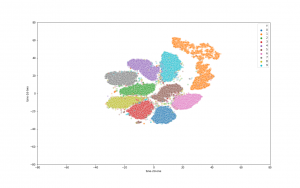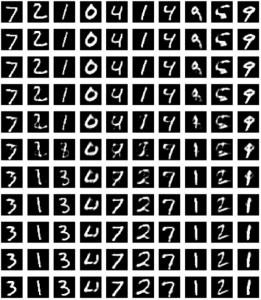## Introduction

Autoencoders provide a powerful framework for learning compressed representations by encoding all of the information needed to reconstruct a data point in a latent code. In some cases, autoencoders can “interpolate”: By decoding the convex combination of the latent codes for two datapoints, the autoencoder can produce an output which semantically mixes characteristics from the datapoints. In this paper, we propose a regularization procedure which encourages interpolated outputs to appear more realistic by fooling a critic network which has been trained to recover the mixing coefficient from interpolated data. We then develop a simple benchmark task where we can quantitatively measure the extent to which various autoencoders can interpolate and show that our regularizer dramatically improves interpolation in this setting. We also demonstrate empirically that our regularizer produces latent codes which are more effective on downstream tasks, suggesting a possible link between interpolation abilities and learning useful representations. – 

The idea comes from the paper “Implementation from paper: Understanding and Improving Interpolation in Autoencoders via an Adversarial Regularizer” (https://arxiv.org/abs/1807.07543), also known as ACAI framework.

Today I will walk through the implementation of this fantastic idea. The implementation is based on tensorflow 2.0 and python 3.6. Let’s start!

## Implementation

First, we need to import some dependency packages.

```import tensorflow as tf
from tensorflow import keras
from tensorflow.keras.datasets import mnist
from tensorflow.keras.layers import Input, Dense, Reshape, Flatten, Dropout, multiply, GaussianNoise
from tensorflow.keras.layers import BatchNormalization, Activation, Embedding, ZeroPadding2D
from tensorflow.keras.layers import MaxPooling2D
from tensorflow.keras.layers import LeakyReLU
from tensorflow.keras.layers import UpSampling2D, Conv2D, Reshape
from tensorflow.keras.layers import Lambda
from tensorflow.keras.models import Sequential, Model
from tensorflow.keras import losses
from tensorflow.keras.utils import to_categorical
from tensorflow.keras.datasets import mnist
import keras.backend as K

import matplotlib.pyplot as plt

import numpy as np
import tqdm
import os

import io
from PIL
import Image
from sklearn.decomposition import PCA
# from sklearn.manifold import TSNE
import seaborn as sns
import pandas as pd
```

Next, we define the overall framework of ACAI, which composes of 3 parts: encoder, decoder and discriminator (also called as critic in the paper).

```class ACAI():
def __init__(self, img_shape=(28,28), latent_dim=32, disc_reg_coef=0.2, ae_reg_coef=0.5, dropout=0.0):
self.latent_dim = latent_dim
self.img_shape = img_shape
self.dropout = dropout
self.disc_reg_coef = disc_reg_coef
self.ae_reg_coef = ae_reg_coef
self.intitializer = tf.keras.initializers.VarianceScaling(
scale=0.2, mode='fan_in', distribution='truncated_normal')
self.initialize_models(self.img_shape, self.latent_dim)

def initialize_models(self, img_shape, latent_dim):
self.encoder = self.build_encoder(img_shape, latent_dim)
self.decoder = self.build_decoder(latent_dim, img_shape)
self.discriminator = self.build_discriminator(latent_dim, img_shape)

img = Input(shape=img_shape)
latent = self.encoder(img)
res_img = self.decoder(latent)

self.autoencoder = Model(img, res_img)
discri_out = self.discriminator(img)

def build_encoder(self, img_shape, latent_dim):
encoder = Sequential(name='encoder')

encoder.summary()
return encoder

def build_decoder(self, latent_dim, img_shape):
decoder = Sequential(name='decoder')

decoder.summary()
return decoder

def build_discriminator(self, latent_dim, img_shape):
discriminator = Sequential(name='discriminator')

discriminator.summary()
return discriminator```

Some utility functions for monitoring the results:

```def make_image_grid(imgs, shape, prefix, save_path, is_show=False):
# Find the implementation in below github repo

def flip_tensor(t):
# Find the implementation in below github repo

def plot_to_image(figure):
# Find the implementation in below github repo

def visualize_latent_space(x, labels, n_clusters, range_lim=(-80, 80), perplexity=40, is_save=False, save_path=None):
# Find the implementation in below github repo```

Next, we define the training worker, which is called at each epoch:

```@tf.function
def train_on_batch(x, y, model: ACAI):
# Randomzie interpolated coefficient alpha
alpha = tf.random.uniform((x.shape, 1), 0, 1)
alpha = 0.5 - tf.abs(alpha - 0.5)  # Make interval [0, 0.5]

# Constructs non-interpolated latent space and decoded input
latent = model.encoder(x, training=True)
res_x = model.decoder(latent, training=True)

ae_loss = tf.reduce_mean(tf.losses.mean_squared_error(tf.reshape(x, (x.shape, -1)), tf.reshape(res_x, (res_x.shape, -1))))

inp_latent = alpha * latent + (1 - alpha) * latent[::-1]
res_x_hat = model.decoder(inp_latent, training=False)

pred_alpha = model.discriminator(res_x_hat, training=True)
# pred_alpha = K.mean(pred_alpha, [1,2,3])
temp = model.discriminator(res_x + model.disc_reg_coef * (x - res_x), training=True)
# temp = K.mean(temp, [1,2,3])
disc_loss_term_1 = tf.reduce_mean(tf.square(pred_alpha - alpha))
disc_loss_term_2 = tf.reduce_mean(tf.square(temp))

reg_ae_loss = model.ae_reg_coef * tf.reduce_mean(tf.square(pred_alpha))

total_ae_loss = ae_loss + reg_ae_loss
total_d_loss = disc_loss_term_1 + disc_loss_term_2

return {
'res_ae_loss': ae_loss,
'reg_ae_loss': reg_ae_loss,
'disc_loss': disc_loss_term_1,
'reg_disc_loss': disc_loss_term_2

}```

Next, we need to define a main training function:

```def train(model: ACAI, x_train, y_train, x_test,
batch_size, epochs=1000, save_interval=200,
save_path='./images'):
n_epochs = tqdm.tqdm_notebook(range(epochs))
total_batches = x_train.shape // batch_size
if not os.path.exists(save_path):
os.makedirs(save_path)
for epoch in n_epochs:
offset = 0
losses = []
random_idx = np.random.randint(0, x_train.shape, x_train.shape)
for batch_iter in range(total_batches):
# Randomly choose each half batch
imgs = x_train[offset:offset + batch_size,::] if (batch_iter < (total_batches - 1)) else x_train[offset:,::]
offset += batch_size

loss = train_on_batch(imgs, None, model)
losses.append(loss)

avg_loss = avg_losses(losses)
# wandb.log({'losses': avg_loss})

if epoch % save_interval == 0 or (epoch == epochs - 1):
sampled_imgs = model.autoencoder(x_test[:100])
res_img = make_image_grid(sampled_imgs.numpy(), (28,28), str(epoch), save_path)

latent = model.encoder(x_train, training=False).numpy()
latent_space_img = visualize_latent_space(latent, y_train, 10, is_save=True, save_path=f'./latent_space/{epoch}.png')
# wandb.log({'res_test_img': [wandb.Image(res_img, caption="Reconstructed images")],
#            'latent_space': [wandb.Image(latent_space_img, caption="Latent space")]})```
```(x_train, y_train), (x_test, y_test) = mnist.load_data()
x_train = x_train.astype(np.float32) / 255.
x_test = x_test.astype(np.float32) / 255.
ann = ACAI(dropout=0.5)
train(model=ann,
x_train=x_train,
y_train=y_train,
x_test=x_test,
batch_size=x_train.shape//4,
epochs=2000,
save_interval=50,
save_path='./images')```

## Results

Some of the result from ACAI after finishing:

First is the visualization of MNIST dataset after encoded by the encoder, we can see that the cluster is well separated and applying downstream tasks on latent space will lead to significant improvement in comparison to raw data (such as clustering, try KMeans and check it out yourself :D).Second is the visualization of interpolation power on latent space:

• Interpolation with alpha values in range [0,1.0] with step 0.1.
• 1st row and final row are source and destination image, respectively.
• Formula:
``````mix_latent = alpha * src_latent + (1 - alpha) * dst_latent
``````We can see that there is a very smooth morphing from the digits at the top row to the digits at the bottom row.

The whole running code is available at github (acai_notebook). It’s your time to play with the paper :D.

## Reference

 David Berthelot, Colin Raffel, Aurko Roy, and Ian Goodfellow. Understanding and improving interpolation in autoencoders via an adversarial regularizer, 2018.

## Pytorch part 1: Introducing Pytorch

Pytorch is a deep learning framework and a scientific computing package
The scientific computing aspect of PyTorch is primarily a result PyTorch’s tensor library and associated tensor operations. That means you can take advantage of Pytorch for many computing tasks, thanks to its supporting tensor operation, without touching deep learning modules.

Important to note that PyTorch tensors and their associated operations are very similar to numpy n-dimensional arrays. A tensor is actually an n-dimensional array.

Pytorch build its library around Object Oriented Programming(OOP) concept. With object oriented programming, we orient our program design and structure around objects. The tensor in Pytorch is presented by the object torch.Tensor which is created from numpy ndarray objects. Two objects share memory. This makes the transition between PyTorch and NumPy very cheap from a performance perspective.

With PyTorch tensors, GPU support is built-in. It’s very easy with PyTorch to move tensors to and from a GPU if we have one installed on our system. Tensors are super important for deep learning and neural networks because they are the data structure that we ultimately use for building and training our neural networks.

The initial release of PyTorch was in October of 2016, and before PyTorch was created, there was and still is, another framework called Torch which is also a machine learning framework but is based on the Lua programming language. The connection between PyTorch and this Lua version, called Torch, exists because many of the developers who maintain the Lua version are the individuals who created PyTorch. And they have been working for Facebook since then till now.

Below are the primary PyTorch modules we’ll be learning about and using as we build neural networks along the way.

## Why use Pytorch for deep learning?

• PyTorch’s design is modern, Pythonic. When we build neural networks with PyTorch, we are super close to programming neural networks from scratch. When we write PyTorch code, we are just writing and extending standard Python classes, and when we debug PyTorch code, we are using the standard Python debugger. It’s written mostly in Python, and only drops into C++ and CUDA code for operations that are performance bottlenecks.
• It is a thin framework, which makes it more likely that PyTorch will be capable of adapting to the rapidly evolving deep learning environment as things change quickly over time.
• Stays out of the way and this makes it so that we can focus on neural networks and less on the actual framework.

## Why PyTorch is great for deep learning research

The reason for this research suitability is that Pytorch use dynamic computational graph, in contrast with tensorfow which uses static computational graph, in order to calculate derivatives.

Computational graphs are used to graph the function operations that occur on tensors inside neural networks. These graphs are then used to compute the derivatives needed to optimize the neural network. Dynamic computational graph means that the graph is generated on the fly as the operations are created. Static graphs that are fully determined before the actual operations occur.